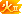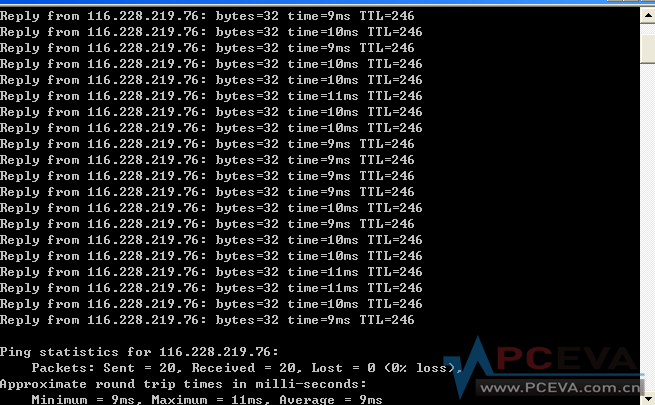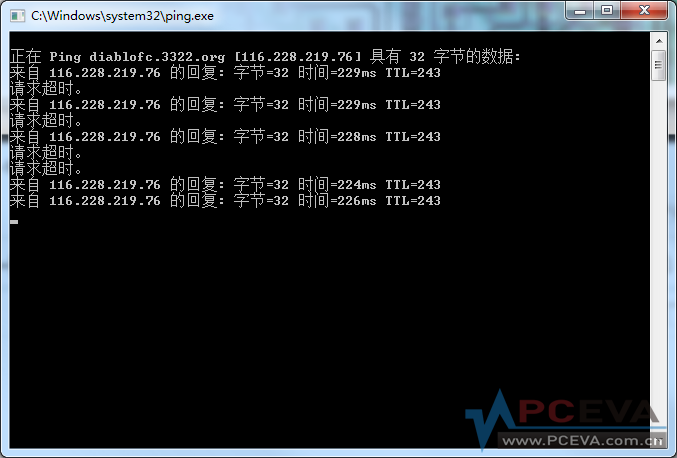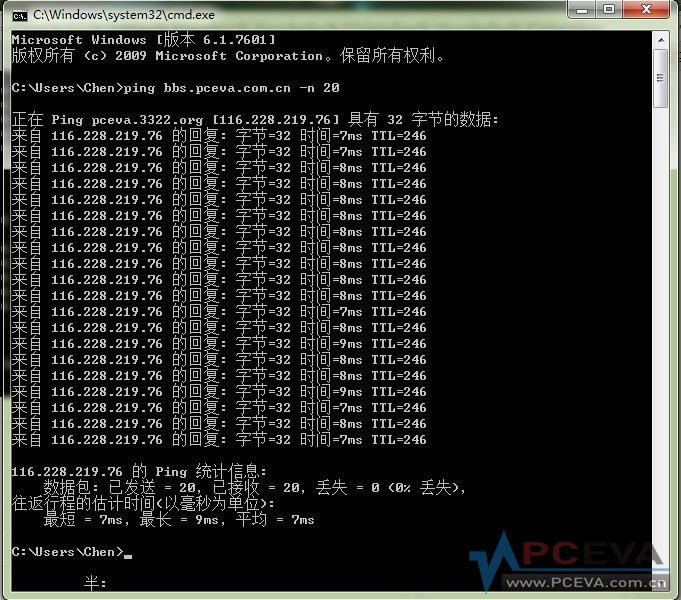# 趁着联通骨干网故障，帮忙做个测试~~~贴出结果的都有分哦[复制链接]
gmx168 发表于 2012-7-11 12:34 | 显示全部楼层 |阅读模式

## 电信 6M### 评分

 本帖最后由 gmx168 于 2012-7-11 12:38 编辑 可能的话，也报一下你的地区、线路种类（电信和联通等等）和带宽。

### 评分gmx168 + 10 徐州电信很给力啊~~~
 本帖最后由 James007ss 于 2012-7-11 12:42 编辑 广州电信 4M ADSL小水管 正在 Ping 116.228.219.76 具有 32 字节的数据: 请求超时。 来自 116.228.219.76 的回复: 字节=32 时间=40ms TTL=246 来自 116.228.219.76 的回复: 字节=32 时间=82ms TTL=246 来自 116.228.219.76 的回复: 字节=32 时间=98ms TTL=246 来自 116.228.219.76 的回复: 字节=32 时间=34ms TTL=246 来自 116.228.219.76 的回复: 字节=32 时间=102ms TTL=246 来自 116.228.219.76 的回复: 字节=32 时间=37ms TTL=246 来自 116.228.219.76 的回复: 字节=32 时间=108ms TTL=246 来自 116.228.219.76 的回复: 字节=32 时间=45ms TTL=246 来自 116.228.219.76 的回复: 字节=32 时间=87ms TTL=246 来自 116.228.219.76 的回复: 字节=32 时间=39ms TTL=246 来自 116.228.219.76 的回复: 字节=32 时间=222ms TTL=246 来自 116.228.219.76 的回复: 字节=32 时间=36ms TTL=246 来自 116.228.219.76 的回复: 字节=32 时间=228ms TTL=246 来自 116.228.219.76 的回复: 字节=32 时间=35ms TTL=246 来自 116.228.219.76 的回复: 字节=32 时间=85ms TTL=246 来自 116.228.219.76 的回复: 字节=32 时间=89ms TTL=246 来自 116.228.219.76 的回复: 字节=32 时间=36ms TTL=246 来自 116.228.219.76 的回复: 字节=32 时间=48ms TTL=246 来自 116.228.219.76 的回复: 字节=32 时间=34ms TTL=246 116.228.219.76 的 Ping 统计信息:     数据包: 已发送 = 20，已接收 = 19，丢失 = 1 (5% 丢失)， 往返行程的估计时间(以毫秒为单位):     最短 = 34ms，最长 = 228ms，平均 = 78ms PS：挂BT中，但BT速度超级不给力

### 评分gmx168 + 10 你是不是在下载啊，延迟跳跃得厉害了.
 James007ss 发表于 2012-7-11 12:41广州电信 4M ADSL小水管 正在 Ping 116.228.219.76 具有 32 字节的数据:福州电信 10M LAN 正在 Ping pceva.3322.org [116.228.219.76] 具有 32 字节的数据: 来自 116.228.219.76 的回复: 字节=32 时间=23ms TTL=245 来自 116.228.219.76 的回复: 字节=32 时间=22ms TTL=245 来自 116.228.219.76 的回复: 字节=32 时间=23ms TTL=245 来自 116.228.219.76 的回复: 字节=32 时间=22ms TTL=245 来自 116.228.219.76 的回复: 字节=32 时间=22ms TTL=245 来自 116.228.219.76 的回复: 字节=32 时间=22ms TTL=245 来自 116.228.219.76 的回复: 字节=32 时间=23ms TTL=245 来自 116.228.219.76 的回复: 字节=32 时间=22ms TTL=245 来自 116.228.219.76 的回复: 字节=32 时间=22ms TTL=245 来自 116.228.219.76 的回复: 字节=32 时间=23ms TTL=245 来自 116.228.219.76 的回复: 字节=32 时间=23ms TTL=245 来自 116.228.219.76 的回复: 字节=32 时间=22ms TTL=245 来自 116.228.219.76 的回复: 字节=32 时间=23ms TTL=245 来自 116.228.219.76 的回复: 字节=32 时间=23ms TTL=245 来自 116.228.219.76 的回复: 字节=32 时间=24ms TTL=245 来自 116.228.219.76 的回复: 字节=32 时间=23ms TTL=245 来自 116.228.219.76 的回复: 字节=32 时间=22ms TTL=245 来自 116.228.219.76 的回复: 字节=32 时间=23ms TTL=245 来自 116.228.219.76 的回复: 字节=32 时间=22ms TTL=245 来自 116.228.219.76 的回复: 字节=32 时间=23ms TTL=245 116.228.219.76 的 Ping 统计信息:     数据包: 已发送 = 20，已接收 = 20，丢失 = 0 (0% 丢失)， 往返行程的估计时间(以毫秒为单位):     最短 = 22ms，最长 = 24ms，平均 = 22ms

### 评分### 评分gmx168 + 10 南北互通依然问题很大啊
 本帖最后由 dubai 于 2012-7-11 13:06 编辑 现在极其的慢。### 点评

 本帖最后由 小猪哥 于 2012-7-11 20:14 编辑 东莞电信 Microsoft Windows [版本 6.1.7601] 版权所有 (c) 2009 Microsoft Corporation。保留所有权利。 正在 Ping pceva.3322.org [116.228.219.76] 具有 32 字节的数据: 来自 116.228.219.76 的回复: 字节=32 时间=31ms TTL=246 来自 116.228.219.76 的回复: 字节=32 时间=30ms TTL=246 来自 116.228.219.76 的回复: 字节=32 时间=30ms TTL=246 来自 116.228.219.76 的回复: 字节=32 时间=30ms TTL=246 来自 116.228.219.76 的回复: 字节=32 时间=29ms TTL=246 来自 116.228.219.76 的回复: 字节=32 时间=32ms TTL=246 来自 116.228.219.76 的回复: 字节=32 时间=31ms TTL=246 来自 116.228.219.76 的回复: 字节=32 时间=31ms TTL=246 来自 116.228.219.76 的回复: 字节=32 时间=29ms TTL=246 来自 116.228.219.76 的回复: 字节=32 时间=30ms TTL=246 来自 116.228.219.76 的回复: 字节=32 时间=30ms TTL=246 来自 116.228.219.76 的回复: 字节=32 时间=30ms TTL=246 来自 116.228.219.76 的回复: 字节=32 时间=30ms TTL=246 来自 116.228.219.76 的回复: 字节=32 时间=30ms TTL=246 来自 116.228.219.76 的回复: 字节=32 时间=33ms TTL=246 来自 116.228.219.76 的回复: 字节=32 时间=33ms TTL=246 来自 116.228.219.76 的回复: 字节=32 时间=32ms TTL=246 来自 116.228.219.76 的回复: 字节=32 时间=32ms TTL=246 来自 116.228.219.76 的回复: 字节=32 时间=32ms TTL=246 来自 116.228.219.76 的回复: 字节=32 时间=31ms TTL=246 116.228.219.76 的 Ping 统计信息:     数据包: 已发送 = 20，已接收 = 20，丢失 = 0 (0% 丢失)， 往返行程的估计时间(以毫秒为单位):     最短 = 29ms，最长 = 33ms，平均 = 30ms

### 点评

luoyong=裸泳，原来桑拿哥好这口呀  发表于 2012-7-11 14:12

### 评分gmx168 + 10 很给力!~~用户名别贴上来吧
 温州电信内部网，网关出口外网估计带宽100M 正在 Ping diablofc.3322.org [116.228.219.76] 具有 32 字节的数据: 来自 116.228.219.76 的回复: 字节=32 时间=12ms TTL=244 来自 116.228.219.76 的回复: 字节=32 时间=12ms TTL=244 来自 116.228.219.76 的回复: 字节=32 时间=32ms TTL=244 来自 116.228.219.76 的回复: 字节=32 时间=21ms TTL=244 来自 116.228.219.76 的回复: 字节=32 时间=13ms TTL=244 来自 116.228.219.76 的回复: 字节=32 时间=14ms TTL=244 来自 116.228.219.76 的回复: 字节=32 时间=12ms TTL=244 来自 116.228.219.76 的回复: 字节=32 时间=47ms TTL=244 来自 116.228.219.76 的回复: 字节=32 时间=12ms TTL=244 来自 116.228.219.76 的回复: 字节=32 时间=13ms TTL=244 来自 116.228.219.76 的回复: 字节=32 时间=12ms TTL=244 来自 116.228.219.76 的回复: 字节=32 时间=12ms TTL=244 来自 116.228.219.76 的回复: 字节=32 时间=11ms TTL=244 来自 116.228.219.76 的回复: 字节=32 时间=13ms TTL=244 来自 116.228.219.76 的回复: 字节=32 时间=12ms TTL=244 来自 116.228.219.76 的回复: 字节=32 时间=12ms TTL=244 来自 116.228.219.76 的回复: 字节=32 时间=11ms TTL=244 来自 116.228.219.76 的回复: 字节=32 时间=11ms TTL=244 来自 116.228.219.76 的回复: 字节=32 时间=28ms TTL=244 来自 116.228.219.76 的回复: 字节=32 时间=13ms TTL=244 116.228.219.76 的 Ping 统计信息:     数据包: 已发送 = 20，已接收 = 20，丢失 = 0 (0% 丢失)， 往返行程的估计时间(以毫秒为单位):     最短 = 11ms，最长 = 47ms，平均 = 16ms

### 评分

 Microsoft Windows [版本 6.1.7601] 版权所有 (c) 2009 Microsoft Corporation。保留所有权利。 C:\Users\hz10>ping bbs.pceva.com.cn -n 20 正在 Ping diablofc.3322.org [116.228.219.76] 具有 32 字节的数据: 来自 116.228.219.76 的回复: 字节=32 时间=185ms TTL=243 来自 116.228.219.76 的回复: 字节=32 时间=191ms TTL=243 来自 116.228.219.76 的回复: 字节=32 时间=187ms TTL=243 来自 116.228.219.76 的回复: 字节=32 时间=192ms TTL=243 来自 116.228.219.76 的回复: 字节=32 时间=182ms TTL=243 来自 116.228.219.76 的回复: 字节=32 时间=177ms TTL=243 来自 116.228.219.76 的回复: 字节=32 时间=184ms TTL=243 来自 116.228.219.76 的回复: 字节=32 时间=172ms TTL=243 来自 116.228.219.76 的回复: 字节=32 时间=166ms TTL=243 来自 116.228.219.76 的回复: 字节=32 时间=169ms TTL=243 来自 116.228.219.76 的回复: 字节=32 时间=168ms TTL=243 来自 116.228.219.76 的回复: 字节=32 时间=171ms TTL=243 来自 116.228.219.76 的回复: 字节=32 时间=167ms TTL=243 来自 116.228.219.76 的回复: 字节=32 时间=165ms TTL=243 来自 116.228.219.76 的回复: 字节=32 时间=158ms TTL=243 来自 116.228.219.76 的回复: 字节=32 时间=165ms TTL=243 来自 116.228.219.76 的回复: 字节=32 时间=168ms TTL=243 来自 116.228.219.76 的回复: 字节=32 时间=163ms TTL=243 来自 116.228.219.76 的回复: 字节=32 时间=157ms TTL=243 来自 116.228.219.76 的回复: 字节=32 时间=159ms TTL=243 116.228.219.76 的 Ping 统计信息:     数据包: 已发送 = 20，已接收 = 20，丢失 = 0 (0% 丢失)， 往返行程的估计时间(以毫秒为单位):     最短 = 157ms，最长 = 192ms，平均 = 172ms C:\Users\hz10> 公司烂宁波联通10M 光纤

### 评分

 广东汕头  公司的电信6M光纤  路由限速 C:\Users\Kyle>ping bbs.pceva.com.cn -n 20 正在 Ping pceva.3322.org [116.228.219.76] 具有 32 字节的数据: 来自 116.228.219.76 的回复: 字节=32 时间=34ms TTL=246 来自 116.228.219.76 的回复: 字节=32 时间=37ms TTL=246 来自 116.228.219.76 的回复: 字节=32 时间=34ms TTL=246 来自 116.228.219.76 的回复: 字节=32 时间=35ms TTL=246 来自 116.228.219.76 的回复: 字节=32 时间=34ms TTL=246 来自 116.228.219.76 的回复: 字节=32 时间=34ms TTL=246 来自 116.228.219.76 的回复: 字节=32 时间=34ms TTL=246 来自 116.228.219.76 的回复: 字节=32 时间=34ms TTL=246 来自 116.228.219.76 的回复: 字节=32 时间=34ms TTL=246 来自 116.228.219.76 的回复: 字节=32 时间=34ms TTL=246 来自 116.228.219.76 的回复: 字节=32 时间=34ms TTL=246 来自 116.228.219.76 的回复: 字节=32 时间=35ms TTL=246 来自 116.228.219.76 的回复: 字节=32 时间=35ms TTL=246 来自 116.228.219.76 的回复: 字节=32 时间=35ms TTL=246 来自 116.228.219.76 的回复: 字节=32 时间=34ms TTL=246 来自 116.228.219.76 的回复: 字节=32 时间=34ms TTL=246 来自 116.228.219.76 的回复: 字节=32 时间=34ms TTL=246 来自 116.228.219.76 的回复: 字节=32 时间=35ms TTL=246 来自 116.228.219.76 的回复: 字节=32 时间=34ms TTL=246 来自 116.228.219.76 的回复: 字节=32 时间=34ms TTL=246 116.228.219.76 的 Ping 统计信息:     数据包: 已发送 = 20，已接收 = 20，丢失 = 0 (0% 丢失)， 往返行程的估计时间(以毫秒为单位):     最短 = 34ms，最长 = 37ms，平均 = 34ms

### 评分

 广州电信DSL 12Mb 正在 Ping pceva.3322.org [116.228.219.76] 具有 32 字节的数据: 来自 116.228.219.76 的回复: 字节=32 时间=64ms TTL=246 来自 116.228.219.76 的回复: 字节=32 时间=61ms TTL=246 来自 116.228.219.76 的回复: 字节=32 时间=71ms TTL=246 来自 116.228.219.76 的回复: 字节=32 时间=61ms TTL=246 来自 116.228.219.76 的回复: 字节=32 时间=57ms TTL=246 来自 116.228.219.76 的回复: 字节=32 时间=68ms TTL=246 请求超时。 来自 116.228.219.76 的回复: 字节=32 时间=108ms TTL=246 来自 116.228.219.76 的回复: 字节=32 时间=57ms TTL=246 来自 116.228.219.76 的回复: 字节=32 时间=58ms TTL=246 来自 116.228.219.76 的回复: 字节=32 时间=59ms TTL=246 来自 116.228.219.76 的回复: 字节=32 时间=213ms TTL=246 来自 116.228.219.76 的回复: 字节=32 时间=60ms TTL=246 来自 116.228.219.76 的回复: 字节=32 时间=60ms TTL=246 来自 116.228.219.76 的回复: 字节=32 时间=67ms TTL=246 来自 116.228.219.76 的回复: 字节=32 时间=160ms TTL=246 来自 116.228.219.76 的回复: 字节=32 时间=285ms TTL=246 来自 116.228.219.76 的回复: 字节=32 时间=168ms TTL=246 来自 116.228.219.76 的回复: 字节=32 时间=145ms TTL=246 来自 116.228.219.76 的回复: 字节=32 时间=100ms TTL=246 116.228.219.76 的 Ping 统计信息:     数据包: 已发送 = 20，已接收 = 19，丢失 = 1 (5% 丢失)， 往返行程的估计时间(以毫秒为单位):     最短 = 57ms，最长 = 285ms，平均 = 101ms

### 评分

 有看视频的和刷微博的
 badaa 发表于 2012-7-11 13:52有看视频的和刷微博的 是啊，adsl就怕上行堵住
 本帖最后由 xiahait 于 2012-7-11 14:13 编辑 青岛网通，2M 企业光线 ping bbs.pceva.com.cn -n 20 正在 Ping diablofc.3322.org [116.228.219.76] 具有 32 字节的数据: 来自 116.228.219.76 的回复: 字节=32 时间=169ms TTL=241 来自 116.228.219.76 的回复: 字节=32 时间=167ms TTL=241 来自 116.228.219.76 的回复: 字节=32 时间=167ms TTL=241 来自 116.228.219.76 的回复: 字节=32 时间=167ms TTL=241 来自 116.228.219.76 的回复: 字节=32 时间=168ms TTL=241 来自 116.228.219.76 的回复: 字节=32 时间=167ms TTL=241 来自 116.228.219.76 的回复: 字节=32 时间=167ms TTL=241 来自 116.228.219.76 的回复: 字节=32 时间=169ms TTL=241 来自 116.228.219.76 的回复: 字节=32 时间=168ms TTL=241 来自 116.228.219.76 的回复: 字节=32 时间=169ms TTL=241 来自 116.228.219.76 的回复: 字节=32 时间=169ms TTL=241 来自 116.228.219.76 的回复: 字节=32 时间=167ms TTL=241 来自 116.228.219.76 的回复: 字节=32 时间=169ms TTL=241 来自 116.228.219.76 的回复: 字节=32 时间=168ms TTL=241 来自 116.228.219.76 的回复: 字节=32 时间=169ms TTL=241 来自 116.228.219.76 的回复: 字节=32 时间=168ms TTL=241 来自 116.228.219.76 的回复: 字节=32 时间=168ms TTL=241 来自 116.228.219.76 的回复: 字节=32 时间=168ms TTL=241 来自 116.228.219.76 的回复: 字节=32 时间=162ms TTL=241 来自 116.228.219.76 的回复: 字节=32 时间=168ms TTL=241 116.228.219.76 的 Ping 统计信息:     数据包: 已发送 = 20，已接收 = 20，丢失 = 0 (0% 丢失)， 往返行程的估计时间(以毫秒为单位):     最短 = 162ms，最长 = 169ms，平均 = 167ms

### 评分gmx168 + 10 还行，在可以接受的范围内
 公司网络  浙江嘉兴  我也不知道是什么线路的。。。### 评分gmx168 + 10 很给力! 一看就是电信线路啦~~~.
 我这边以前秒开，现在不给力呀，老湿
 上海移动2g网络，android终端模拟器ping的，仅供参考 \$ ping -c 10 bbs.pceva.com.cn PING diablofc.3322.org (116.228.219.76) 56(84) bytes of data.                   64 bytes from 116.228.219.76: icmp_seq=1 ttl=239 time=353 ms                    64 bytes from 116.228.219.76: icmp_seq=2 ttl=239 time=236 ms                    64 bytes from 116.228.219.76: icmp_seq=3 ttl=239 time=496 ms                    64 bytes from 116.228.219.76: icmp_seq=4 ttl=239 time=377 ms 64 bytes from 116.228.219.76: icmp_seq=5 ttl=239 time=337 ms 64 bytes from 116.228.219.76: icmp_seq=6 ttl=239 time=253 ms 64 bytes from 116.228.219.76: icmp_seq=7 ttl=239 time=414 ms 64 bytes from 116.228.219.76: icmp_seq=8 ttl=239 time=372 ms 64 bytes from 116.228.219.76: icmp_seq=9 ttl=239 time=1370 ms 64 bytes from 116.228.219.76: icmp_seq=10 ttl=239 time=386 ms --- diablofc.3322.org ping statistics --- 10 packets transmitted, 10 received, 0% packet loss, time 9008ms rtt min/avg/max/mdev = 236.160/459.895/1370.010/311.498 ms, pipe 2 \$

### 评分gmx168 + 10 移动就算了吧，好不了的。
 您需要登录后才可以回帖 登录 | 注册 本版积分规则 回帖并转播 回帖后跳转到最后一页